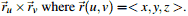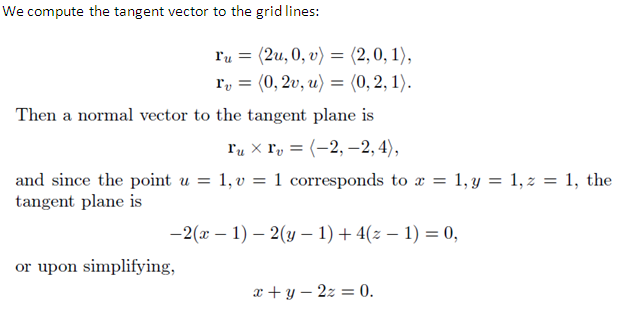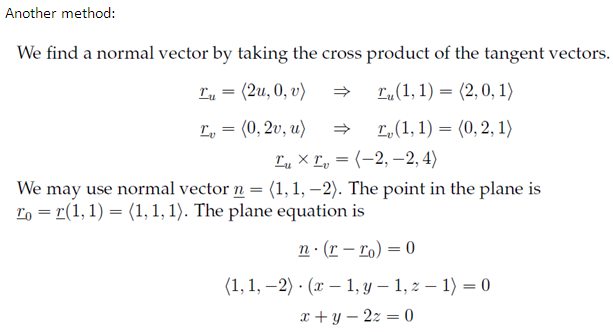" />

# Find the equation of the tangent plane to x = u2, y = v2 and z = (uv) at the point (1, 1, 1). The normal vector for the plane can be found by computin

Publish On: 2019-03-15

Total Post: 416

# Mjay Jollay

Total Post: 0

## ANS: Find the equation of the tangent plane to x = u2, y = v2 and z = (uv) at the point (1, 1, 1). The normal vector for the plane can be found by computing